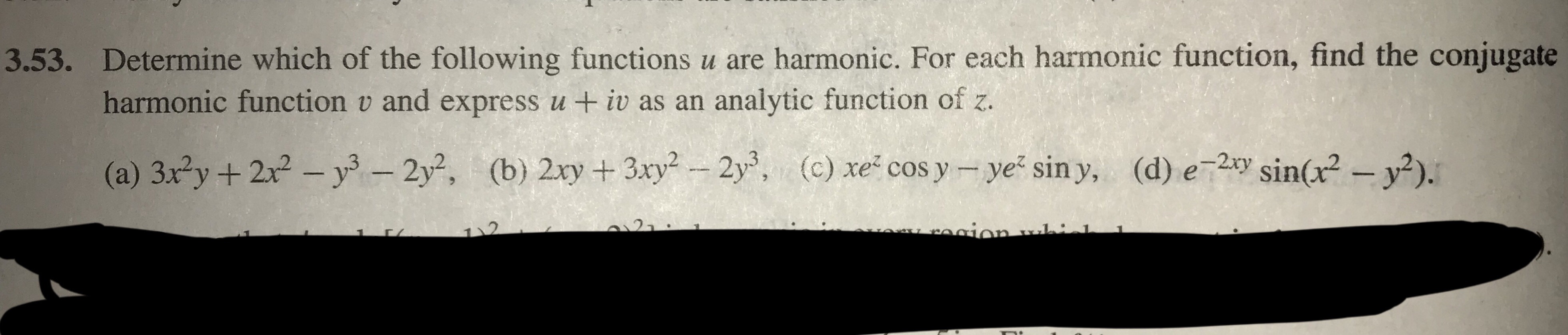# Determine which of the following functions u are harmonic. For each harmonic function, find the conjugateharmonic function v and express u +iv as an analytic function of z.3.53.(b) 2xy + 3xy2-2y,(a) 3x2y + 2x2-уз-2y2,(d) e_2xy Sin(x2-y2).(c) xe-cosy-yez siny,

Question
137 views

z is a complex variable

z=x+iy

question attached in photohelp_outlineImage TranscriptioncloseDetermine which of the following functions u are harmonic. For each harmonic function, find the conjugate harmonic function v and express u +iv as an analytic function of z. 3.53. (b) 2xy + 3xy2-2y, (a) 3x2y + 2x2-уз-2y2, (d) e_2xy Sin(x2-y2). (c) xe-cosy-yez siny, fullscreen
check_circle

Step 1

As per norms, the first three parts A, B and C are solved.To test if the given function is harmonic and find the harmonic conjugate in that case

Step 2

Basic definitions and Cauchy Riemann equations

Step 3

a) given function u is harmonic Remains ...

### Want to see the full answer?

See Solution

#### Want to see this answer and more?

Solutions are written by subject experts who are available 24/7. Questions are typically answered within 1 hour.*

See Solution
*Response times may vary by subject and question.
Tagged in